## Polynomial Remainder Theorem

If the Coefficients of the Polynomial(1)

are specified to be Integers, then integral Roots must have a Numerator which is a factor of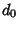and a Denominator which is a factor of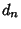(with either sign possible). This follows since a Polynomial of Orderwith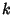integral Roots can be expressed as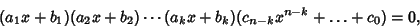(2)

where the Roots are,, ..., and. Factoring out the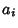s,(3)

Now, multiplying through,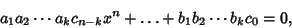(4)

where we have not bothered with the other terms. Since the first and last Coefficients areand, all the integral roots of (1) are of the form [factors of]/[factors of].